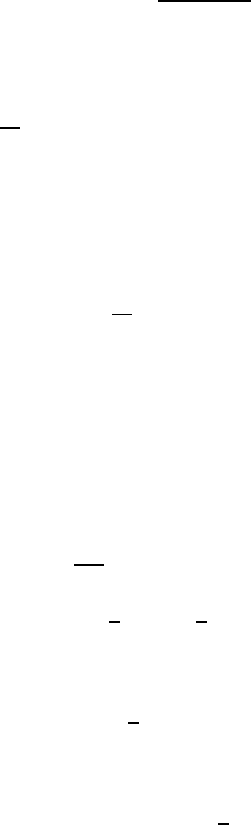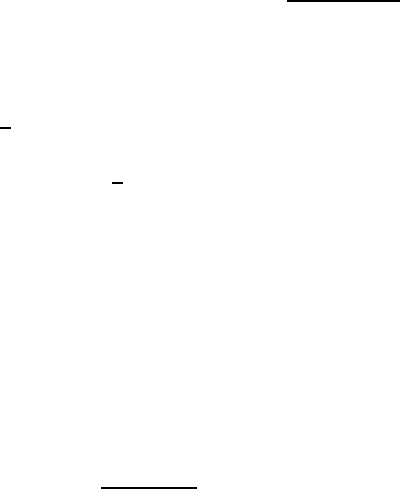Study Guides (390,000)
US (220,000)
UC-Irvine (3,000)
MATH (600)
MATH 2B (100)
All (60)
Midterm

# MATH 2B Study Guide - Midterm Guide: Partial Fraction Decomposition, Indeterminate FormPremium

Department
Mathematics
Course Code
MATH 2B
Professor
All
Study Guide
Midterm

This preview shows page 1. to view the full 5 pages of the document.Section 7.5, 7.8 - Improper Integrals
Reviewing the definition of an integral
() = 

()
What does this mean?
The integral of the function ()on the interval from to ,
Is the limit of the sum of () multiplied by the change of x
Whenever we have some number in [,] that is NOT in the domain of (), the
integral is said to be improper
Example #1

We know this is improper because of the boundary of
How do we solve this?
Think about replacing with a different variable



We can take the limit as T goes to
And then replace with T in the integral to make it possible to solve for
After taking the integral, we would then take the limit





 ( 1  )


(
)
Then we can take the limit after simplifying

 1
The limit as 1 approaches is just 1
The limit as
approaches is 0
1 0 = 1

Unlock to view full version

Subscribers Only

Only page 1 are available for preview. Some parts have been intentionally blurred.

Subscribers OnlyBecause the limit is a definitive number, and not an answer such as +,
or , it is said to convergent
Example #2





||( 1  )

|| |1|

|| 0
The limit as T approaches on || is +
Because the answer is NOT a definitive number, the limit is said to be
divergent
Example #3
 



From here, we notice that we have a not so easy integral to evaluate
Use integration by parts
= ,  = (1)
 = , =

(  0)


(  0) (  0)

(0) ()


This is an indeterminate form
So to solve this problem , we will have to use L’Hopital’s Rule